### Home > A2C > Chapter Ch11 > Lesson 11.3.2 > Problem11-142

11-142.
1. Change each of the following equations to graphing form, identify the conic, and sketch the graph. Homework Help ✎

1. 4x2y2 − 24x − 10y = 5

2. x2 + y + 10x − 4y = −13

3. 4x2 + 9y2 + 24x − 36y = −36

4. 3x2 − 12x − y = −17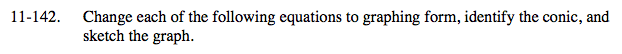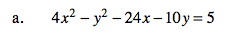4(x2 − 6x + 9) − (y2 + 10y + 25) = 5 + 36 − 25

4(x − 3)2 − (y + 5)2 = 16

$\frac{(x-3)^2}{4}-\frac{(y+5)^2}{16}=1$

This is a hyperbola.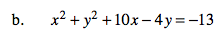See part (a).

(x + 5)2 + (y − 2)2 = 16
This is a circle.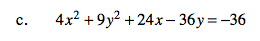See part (a).

$\frac{(x+3)^2}{9}+\frac{(y-2)^2}{4}=1$

This is an ellipse.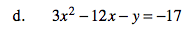Set the equation equal to y and complete the square for x.

y = 3(x − 2)2 + 5
This is a parabola.